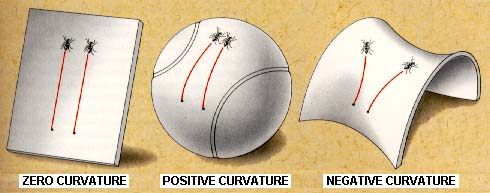# Differential GeometryFrom "A Journey into Gravity and Spacetime" by Wheeler.

### Course Info

 Instructor: Facundo Mémoli,   m e m o l i @ m a t h . o s u . e d u Course code: Math 6702 -- Spring 2015 Times: MWF -- 1.50--2.45 Location: Dulles Hall 020. Map Topics: Riemannian manifolds, distance, volume; covariant derivatives, Levi-Civita connection; geodesics, curvatures: sectional, Ricci, scalar; Jacobi fields, conjugate points; Rauch comparison, Hopf-Rinow, Hadamard, Preissman's and Berger's Sphere Theorems. Other topics: Spectral geometry, families of manifolds with bounded geometry. Assessment: TBA. Prerequisites: Some degree of familiarity with differentiable manifolds and differential geometry of curves and surfaces. See below for background reading.

### Lectures and resources

 Books: The book by Gallot, Hulin & LaFontaine ([GHL] from now on) is a good resource. I may also use parts of Sakai's book ([S] from now on), and Petersen's book ([P] from now on). The book by Berger "A panoramic view of Riemannian Geometry" ([B]) is a very good read. The following paper by Petersen is a very good summary of some global results in RG: "Aspects of global Riemannian Geometry" ([AGRG]). Assumed background. To read on your own: Chapter 1 of [GHL] and Chapters 1 to 4 of Do Carmo's Differential Geometry of Curves and Surfaces. Lecture 1 (Jan. 12th). $(M,g^M)\in\mathcal{R}$ and maps $F:M\rightarrow N$ between RMs. Metric tensors in \mathbb{R}^n. Riemannian metrics on manifolds. Existence of a Riemannian metric. Some examples. Lecture 2 (Jan 14th). Length of curves. RMs as metric spaces. Lecture 3 (Jan. 16th). Energy of curves, relationship to Length. Isometries of metric spaces vs. Riemannian isometries -- the Myers-Steenrod theorem. Lecture 4 (Jan. 21st). Connections. The Levi-Civita connection. Lecture 5 (Jan. 23rd). Expression in local coordinates. Christoffel symbols. Covariant derivative along a curve. Parallel translation. Gradient. Lecture 6 (Jan. 26th) Covariant derivative of tensors. Hessian of a function. Laplace-Beltrami operator. The flat case. Lecture 7 (Jan. 28th). Geodesics. Geodesic equation. Lecture 8 (Jan. 30th). The exponential map. Normal coordinates. Gauss Lemma. Lecture 9 (Feb. 2nd). Student presentation: Sunhyuk Lim -- Systolic Geometry. Lecture 10 (Feb. 4th). Student presentation: Corey Staten -- The Myers-Steenrod theorem. Lecture 11 (Feb. 6th). Student presentation: Irfan Glogic -- Classification of Flat Tori. Lecture 12 (Feb. 9th) First variation of length. Geodesic completeness. Hopf-Rinow theorem. Lecture 13 (Feb. 11th) The Riemann curvature Tensor. Lecture 14 (Feb. 13th) Sectional, Ricci, and Scalar curvature. Lecture 15 (Feb. 16th) Spaces of constant curvature. Lecture 16 (Feb. 18th) First and second Variation of Energy and Length. Lecture 17 (Feb. 20th) Jacobi fields (I). Lecture 18 (Feb. 23rd) Jacobi fields (II). Geodesic variations. Lecture 19 (Feb. 25th) Geodesic variations. Taylor Expansion of the metric. Lecture 20 (Feb. 27th). Interpretation of Jacobi fields. Conjugate points. Lecture 21 (Mar. 2nd). Student presentation: Jimmy Voss -- Geodesics on $P^n(\mathbb{C})$. Lecture 22 (Mar. 4th). Student presentation: Waylon Chen -- Metrics on Lie Groups. Lecture 23 (Mar. 6th). Student presentation: Samir Chowdhury -- Model spaces: their geodesics and sectional curvatures. Lecture 24 (Mar. 9th). Gauss Lemma and applications. Lecture 25 (Mar. 11th). The index form. Jacobi theorems. Lecture 26 (Mar. 13th). Bonnet-Myers theorem. Lecture 27 (Mar. 23rd). Hadamard theorem. Morse-Schoenberg theorem. Rauch I Comparison Theorem for Jacobi fields. Lecture 28 (Mar. 25th). Statement of Volume comparison theorems. Cheng's maximal diameter theorem. Lecture 29 (Mar. 27th). Rauch II. Lecture 30 (Mar. 30th). Metric tensor on manifolds of constant curvature. Lecture 31 (Apr. 1st). Volume of metric balls in manifolds of constant curvature. Multidimensional variations. Lecture 32 (Apr. 3rd). Matricial Jacobi equation. Volume of metric balls. Lecture 33 (Apr. 6th). Bishop-Gunther theorem. Lecture 34 (Apr. 8th). Bishop volume comparison theorem. Lecture 35 (Apr. 10th). Gromov's relative volume comparison theorem. Gromov's precompactness theorem. Lecture 36 (Apr. 13th). Gromov's precompactness theorem and the GH distance. Lecture 37 (Apr. 15th).. Lecture 38 (Apr. 17th).. Lecture 39 (Apr. 20th).. Lecture 40 (Apr. 22th).. Lecture 41 (Apr. 24th).. Lecture 42 (Apr. 27th)..